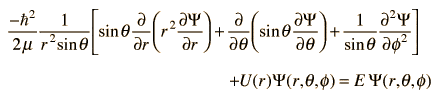# Show that this function satisfies Schrödinger equation for hydogen?

• Mixer

## Homework Statement

Show that function

Y = C sin θ (5cos2θ - 1 )ei$\phi$

satisfies Scrhödinger equation for hydrogen?

## Homework Equations## The Attempt at a Solution

I derivated the required elements of the equation but ended up to some messy equation with lots of sines and cosines here and there.. I checked the derivations with WolframAlpha and got the same result so they are ( I hope) correct. What exactly am I supposed to do?

You are supposed to simplify the mess - specifically you are trying to show that the whole mess can be simplified to a constant multiplied by the original wavefunction.

Welcome to wave mechanics xD